# Intelligent Charger for 9V NiMH Rechargeable Batteries V1

Regulated smart constant current charger for 9V NiMH rechargeable batteries.

BeginnerFull instructions provided2 hours16,859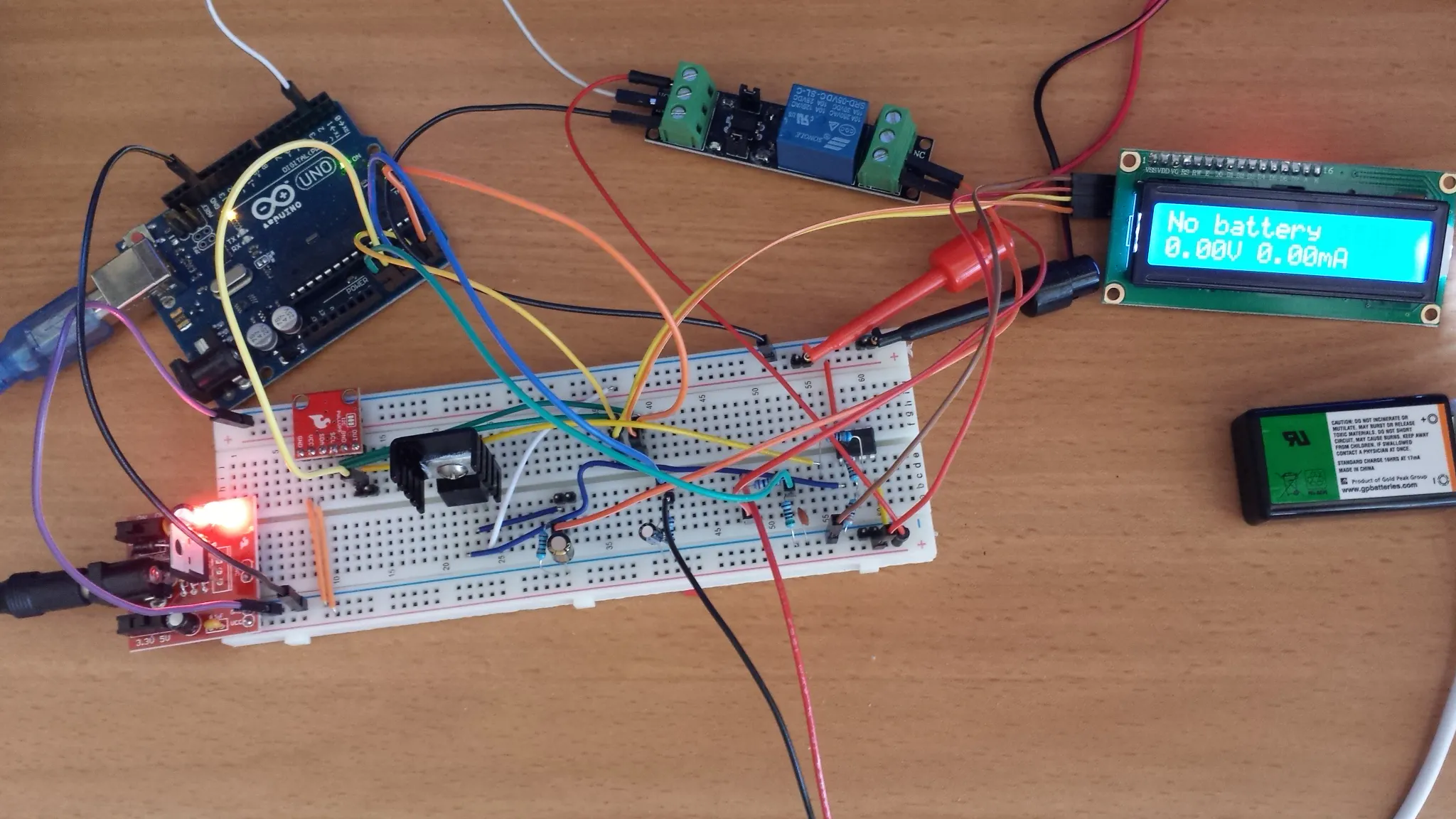## Things used in this project

### Hardware componentsArduino UNO & Genuino UNO
×1
 SparkFun I2C DAC Breakout - MCP4725
×1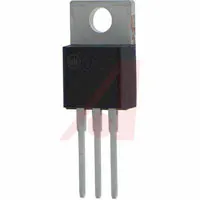×1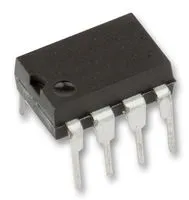Texas Instruments General Purpose Dual Op-Amp
×1
 Resistor 6.8k ohm
×2
 Resistor 3.3k ohm
×1
 Resistor 5.1k ohm
×1Resistor 1k ohm
×1
 Resistor 10 ohm
×1
 Relay (generic)
×1
 Resistor 20k ohm
×1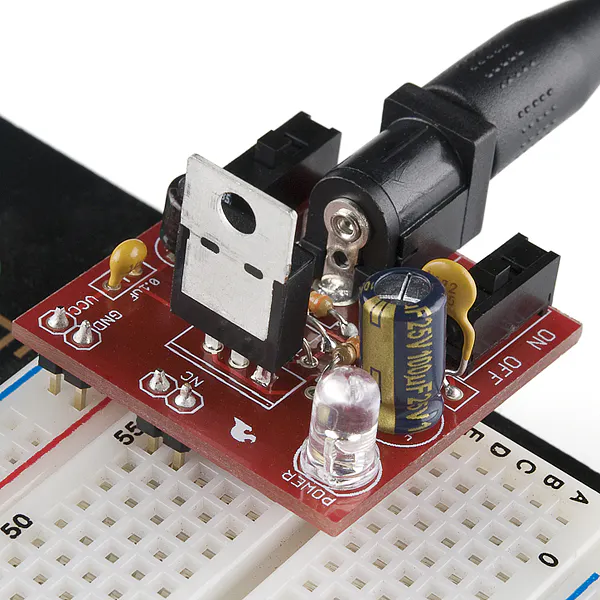×1
 Power supply 17V
×1
 YwRobot I2C SERIAL LCD 1602 MODULE
×1

## Schematics

### Schematic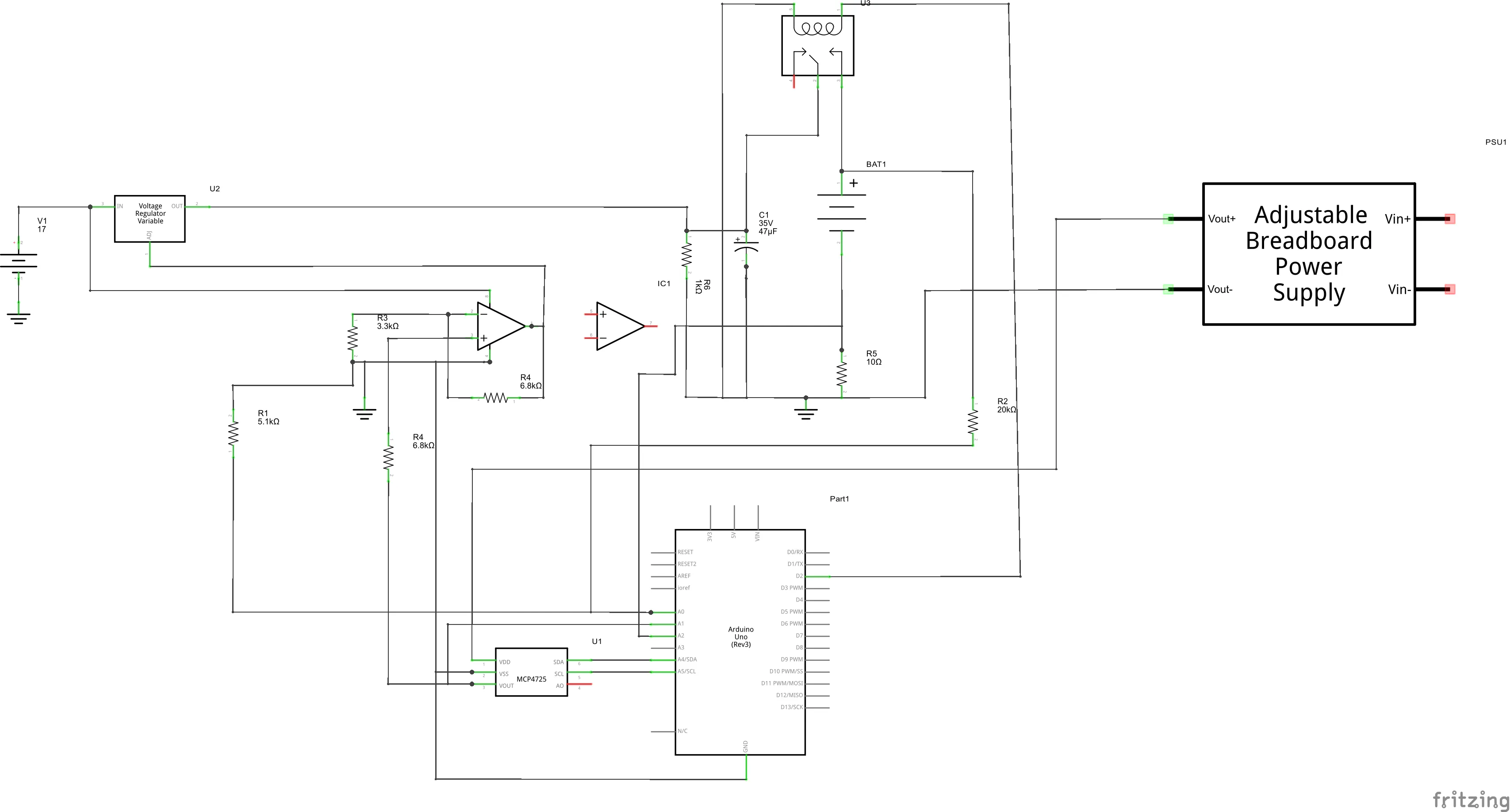## Code

### charger.ino

Arduino
Main file
```//THis for charging 9V NiMH battery
//Battery can have have 6,7 or 8 1.25V cells
//This makes nominal voltage between 7.5 and 10V

#include <Wire.h>
#include <LiquidCrystal_I2C.h>
#include <TimeLib.h>
#include <TimerOne.h>
#include <elapsedMillis.h>

LiquidCrystal_I2C lcd(0x27, 2, 1, 0, 4, 5, 6, 7, 3, POSITIVE);  // set the LCD address to 0x27 for a 16 chars and 2 line display

//Hardware defenitions
#define DAC_REF_VOLTAGE 5.0
#define CHARGE_RELAY_PIN 2
#define DISCONNECT_CHARGE_RELAY HIGH
#define CONNECT_CHARGE_RELAY LOW
#define CURRENT_SENSING_SESISTOR_PIN A2
#define CURRENT_SENSING_SESISTOR 10.2 //OHm
#define BATTERY_VOLTAGE_PIN A0
#define R1 5090 //Low side resistor of voltage sensing divider
#define R2 19910//High side resistor of voltage sensing divider
#define ADC_REF_VOLTAGE 4.89 //Arduino real supply voltage or AREF voltage for proper ADC to voltage translation
#define R3 3300.0
#define R4 6800.0
#define AMP_GAIN (1+R4/R3)

//Various definitions
//#define MINIMUM_VOLTAGE 8.1 //Minimum voltage after regulator
#define BATTERY_GOOD_VOLTAGE_THRESHOLD 7.0
#define MAXIMIM_ALLOWED_CURRENT 200 //Maximum allowed battery current mA (hard cut-off)
#define MAXIMUM_BATTERY_VOLTAGE 15.0 //Maximum allowed battery voltage V (hard cut-off)
#define VOLTAGE_STEP 0.001 //Step for voltage regulation
#define POINTS_FOR_AVERAGING 100 //How many points taken for averaging
#define MEASURE2MEASURE_US 5000 //Time between measurments in microseconds (need be more then 200 becouse of two analog reads)
#define VOLTAGE_LOG_TIME_MIN 30.0 //Time in minutes to save voltage for dV/dT cutoff
#define MINUTES_TO_LOG 5
#define TRICKLE_CURRENT_RATIO 40

//Charger states
#define INITIALIZATION 0
#define NO_BATTERY 5
#define BATTERY_CONNECTED 10
#define BATTERY_VOLTAGE_LOW 15
#define CURRENT_RAMP_UP 20
#define CHARGING 30
#define CURRENT_RAMP_DOWN 32
#define TRICKLE 35
#define END_OF_TRICKLE 37
#define UNEXPECTED_CURRENT_FALL 40
#define UNABLE_TO_REACH_CURRENT 60
#define FULLY_CHARGED 70
#define CURRENT_TOO_HIGH 80
#define REGULATION_FAILED 90
#define OVERCURRENT 100
#define OVERVOLTAGE 101
#define FINAL_STATE 200

///
long unsigned int voltage_sum,current_sum;//Sum of averaging
float regulator_voltage_code,resistor_voltage,csr_voltage_code,regulator_voltage,current_mA,battery_voltage;//Measurments
float tmp_resistor_voltage,tmp_current_mA,tmp_regulator_voltage,tmp_battery_voltage;
int i,j,charger_state;
short unsigned int last_second,lcd_last_second,log_last_second;
String lcd_last_string1,lcd_last_string2;
String empty_string="";
String msg,eoc_line1,eoc_line2;
unsigned char sec_index,min_index;
//long long int charging_started;
float sec_log,min_log[MINUTES_TO_LOG],last_blf;
float trickle_current_mA;
int total_minutes_average=0;

elapsedMillis ChargingTimeMillis,TrickleChargingTimeMillis;

float wanted_dac_voltage=0;
float last_dac_voltage=0;

//Messages
const char msg_battery_detected[] PROGMEM 		="Battery detected";
const char msg_no_battery[] PROGMEM				="No battery";
const char msg_battery_ok[] PROGMEM				="Battery ok";
const char msg_voltage_too_low[] PROGMEM		="Battery voltage too low";
const char msg_voltage_too_low_short[] PROGMEM	="V Battery low";
const char msg_ramp_up[] PROGMEM				="Ramp up";
const char msg_charging[] PROGMEM				="Charging";
const char msg_space[] PROGMEM					=" ";
const char msg_ramp_down[] PROGMEM				="Ramp down";
const char msg_trickle_charge[] PROGMEM			="Trickle charge";
const char msg_no_current[] PROGMEM				="No current";
const char msg_current_unreachable[] PROGMEM	="I unreachable";
const char msg_current_unreachable_long[] PROGMEM	="Unable to reach desired current";
const char msg_completed[] PROGMEM				="Completed";
const char msg_charge[] PROGMEM					="Charge";
const char msg_high_current[] PROGMEM			="High current";
const char msg_regulation_fault[] PROGMEM		="Regulation fault";
const char msg_overcurrent[] PROGMEM			="Current too high";
const char msg_overvoltage[] PROGMEM			="Voltage too high";
const char msg_trickle_completed[] PROGMEM		="Trickle finished";

//*************************** Charging parameters *****************************************
//*****************************************************************************************
float target_current_mA=30;				//Charging current mA
float battery_nominal_capacity_mA=170;	//Nominal capacity of battery mA
float max_time_for_trickle_charge=6;  //Maximum trickle charge time in minutes
//*****************************************************************************************
//*****************************************************************************************

struct mytime {
unsigned char hours;
unsigned char minutes;
unsigned int total_minutes;
} elapsed_time;

void setup() {
pinMode(CHARGE_RELAY_PIN, OUTPUT);
disconnect_charging_circuit();	//Disconnect charger ftom battery
Wire.begin();//I2C
dac_write_voltage(0);//Make sure lower possible current set

Serial.begin(115200);
last_second=second();
lcd_last_second=second();
log_last_second=second();

Timer1.initialize(MEASURE2MEASURE_US); //Will use to measure voltage and current of battery (microseconds)
averaging_index=0;
sec_index=0;
min_index=0;
charger_state=0;//Initial state of state machine
wanted_dac_voltage=0;//Make shure minimum voltage outout
last_blf=1.0;
trickle_current_mA=battery_nominal_capacity_mA/TRICKLE_CURRENT_RATIO;
//ChargingTimeMillis=0;
//LCD
lcd.begin(16,2);
lcd.backlight();
lcd.clear();
update_lcd(F("Power up..."),empty_string);
delay(1000);
}

float log_battery_voltage(){
//Log only once per second
if (log_last_second==second()) return last_blf;
else log_last_second=second();

sec_log[sec_index]=battery_voltage;
if (sec_index<59) {
sec_index++;
}
else {//If one minute logged
//Calculate avetage per minute
if (min_index>=MINUTES_TO_LOG) min_index=0;

sec_index=0;
float sum_v=0;
for (i=0;i<60;i++){
sum_v+=sec_log[i];
}
float min_average=sum_v/60.0;

for(i=1;i<MINUTES_TO_LOG;i++) min_log[i-1]=min_log[i];

min_log[MINUTES_TO_LOG-1]=min_average;//Save minute average

//Serial.print(min_index);
//Serial.print(" ");
//Serial.print(min_average);
//Serial.print(" arr: ");
//Serial.print(min_log);
//Serial.print(" ");
//Serial.print(min_log);
//Serial.print(" ");
//Serial.print(min_log);
//Serial.print(" ");
//Serial.print(min_log);
//Serial.print(" ");
//Serial.print(min_log);
last_blf=best_linear_fit(min_log);
//Serial.print("Slope ");
//Serial.println(last_blf);
min_index++;
total_minutes_average++;
}
return last_blf;
}
```

### main.ino

Arduino
```void loop() {
String msg1;
switch(charger_state){
case INITIALIZATION://Initial state
disconnect_charging_circuit();//Make shure relay disconnected
dac_write_voltage(0);//Make sure lower possible current set
wanted_dac_voltage=0;//Make shure minimum voltage outout
delay(100);
if (battery_voltage>0.1) {
charger_state=BATTERY_CONNECTED;//Battery detected
update_lcd(M2S(msg_battery_detected),empty_string);
Serial.println(M2S(msg_battery_detected));
delay(2000);
}
else {//No battery
Serial.println(M2S(msg_no_battery));
update_lcd(M2S(msg_no_battery),construct_status_string());
charger_state=NO_BATTERY;//Battery detected
delay(1000);
}
break;
case NO_BATTERY://No battery
if (battery_voltage>0.1) {
charger_state=BATTERY_CONNECTED;//Battery detected
Serial.println(M2S(msg_battery_detected));
update_lcd(M2S(msg_battery_detected),construct_status_string());
delay(1500);
}
else{
//If no battery stay in this state
update_lcd(M2S(msg_no_battery),construct_status_string());
delay(1100);
}
break;
case BATTERY_CONNECTED://Battery connected
dac_write_voltage(0);//Make sure lower possible current set
wanted_dac_voltage=0;
delay(100);
if (battery_voltage>BATTERY_GOOD_VOLTAGE_THRESHOLD){
charger_state=CURRENT_RAMP_UP;//Start charging current rampup
//snprintf(welcome, sizeof(welcome),"Firmware:V%d.%d%d",ver,ver2,ver3);
update_lcd(M2S(msg_battery_ok),construct_status_string());
Serial.println(M2S(msg_battery_ok));
delay(2000);
wanted_dac_voltage=get_approximated_dac_voltage(battery_voltage);//Set needed regulator voltage
//Serial.println(get_approximated_dac_voltage(battery_voltage));
connect_charging_circuit();
delay(200);
}
else {
charger_state=BATTERY_VOLTAGE_LOW;//Battery voltage too low
Serial.println(M2S(msg_voltage_too_low));
update_lcd(M2S(msg_voltage_too_low_short),construct_status_string());
delay(1000);
}
break;
case BATTERY_VOLTAGE_LOW://Battery voltage too low
update_lcd(M2S(msg_voltage_too_low_short),construct_status_string());
Serial.println(M2S(msg_voltage_too_low));
charger_state=FINAL_STATE;//Halt
break;
case CURRENT_RAMP_UP:///Current rampup
//if (current_mA<1.0) charger_state=40;//Current unexpectedly fell
update_lcd(M2S(msg_ramp_up),construct_status_string());
delay(50);
if (current_mA<target_current_mA) {
if (wanted_dac_voltage<4.99) {
wanted_dac_voltage=wanted_dac_voltage+VOLTAGE_STEP;
dac_write_voltage(wanted_dac_voltage);
}
else charger_state=UNABLE_TO_REACH_CURRENT;//Unable to reach desired current
}
else {
charger_state=CHARGING;//Charging
ChargingTimeMillis=0;//Reset counter
}
break;
case CHARGING://Charging
delay(200);
if (current_mA<1.0) charger_state=UNEXPECTED_CURRENT_FALL;//Current unexpectedly fell
if (battery_voltage>MAXIMUM_BATTERY_VOLTAGE ) charger_state=OVERVOLTAGE;//Overvoltage
if (abs(current_mA-target_current_mA)>0.2){//If current deviated from target
if (current_mA<target_current_mA) {//If current less theb target
if (wanted_dac_voltage<4.99) wanted_dac_voltage=wanted_dac_voltage+VOLTAGE_STEP;
else charger_state=UNABLE_TO_REACH_CURRENT;//Unable to reach desired current
}
else {
if (wanted_dac_voltage>0.01) wanted_dac_voltage=wanted_dac_voltage-VOLTAGE_STEP;
else charger_state=CURRENT_TOO_HIGH;//Current too high, unable to lower
}
}
if (abs(current_mA-target_current_mA)>5){//Regulation failed, difference too high
charger_state=REGULATION_FAILED;//Regulation error, difference too high
}
dac_write_voltage(wanted_dac_voltage);
if (total_minutes_average<MINUTES_TO_LOG) msg =String(M2S(msg_charging)+M2S(msg_space)+String("---"));
else msg =String(M2S(msg_charging)+M2S(msg_space)+String(last_blf,3));
//snprintf(myString,sizeof(myString), "Alarm %d is set M-F at %d:%02d AM",alrmNum+1,myhours,alrmMM[alrmNum]);
update_lcd(msg,construct_status_string());
log_battery_voltage();
if (last_blf<0) charger_state=FULLY_CHARGED;//If negative dV/dt detected, battery fully charged
break;
case CURRENT_RAMP_DOWN:///Current rampup
//if (current_mA<1.0) charger_state=40;//Current unexpectedly fell
update_lcd(M2S(msg_ramp_down),construct_status_string());
Serial.println(M2S(msg_ramp_down));
delay(50);
if (current_mA>trickle_current_mA) {
if (wanted_dac_voltage>VOLTAGE_STEP) {
wanted_dac_voltage=wanted_dac_voltage-VOLTAGE_STEP;
dac_write_voltage(wanted_dac_voltage);
}
else charger_state=CURRENT_TOO_HIGH;//Unable to reduce current to trickle rate
}
else {
charger_state=TRICKLE;//Charging
TrickleChargingTimeMillis=0;
Serial.println(M2S(msg_trickle_charge));
}
break;
case TRICKLE://Charging
delay(200);
if (current_mA<0.2) charger_state=UNEXPECTED_CURRENT_FALL;//Current unexpectedly fell
if (battery_voltage>MAXIMUM_BATTERY_VOLTAGE ) charger_state=OVERVOLTAGE;//Overvoltage
if (abs(current_mA-trickle_current_mA)>0.2){//If current deviated from target
if (current_mA<trickle_current_mA) {//If current less theb target
if (wanted_dac_voltage<4.99) wanted_dac_voltage=wanted_dac_voltage+VOLTAGE_STEP;
else charger_state=UNABLE_TO_REACH_CURRENT;//Unable to reach desired current
}
else {
if (wanted_dac_voltage>0.01) wanted_dac_voltage=wanted_dac_voltage-VOLTAGE_STEP;
else charger_state=CURRENT_TOO_HIGH;//Current too high, unable to lower
}
}
if (abs(current_mA-trickle_current_mA)>5){//Regulation failed, difference too high
charger_state=REGULATION_FAILED;//Regulation error, difference too high
}
dac_write_voltage(wanted_dac_voltage);
//if (total_minutes_average<MINUTES_TO_LOG) msg =String(F("Charging "))+String("---");
//else msg =String(F("Charging "))+String(last_blf,3);
//Serial.println(msg_trickle_charge);
//snprintf(myString,sizeof(myString), "Alarm %d is set M-F at %d:%02d AM",alrmNum+1,myhours,alrmMM[alrmNum]);
if ((second()%8)<4) update_lcd(M2S(msg_trickle_charge),construct_status_string());
else update_lcd(eoc_line1,eoc_line2);
//log_battery_voltage();
//if (last_blf<0) charger_state=FULLY_CHARGED;//If negative dV/dt detected, battery fully charged
elapsed_time=mills2time(TrickleChargingTimeMillis);
if (elapsed_time.total_minutes>max_time_for_trickle_charge) {//Maximum allowed trickle charge
update_lcd(eoc_line1,eoc_line2);
charger_state=END_OF_TRICKLE;//Halt
disconnect_charging_circuit();	//Disconnect charger from battery
}
break;
case END_OF_TRICKLE:
if ((second()%8)<4) update_lcd(M2S(msg_trickle_completed),construct_status_string());
else update_lcd(eoc_line1,eoc_line2);
break;
case UNEXPECTED_CURRENT_FALL://Current unexpectedly fell
Serial.println(F("Current unexpectedly fell"));
disconnect_charging_circuit();
wanted_dac_voltage=0;
update_lcd(M2S(msg_no_current),construct_status_string());
charger_state=FINAL_STATE;//Halt
delay(1000);
break;
case UNABLE_TO_REACH_CURRENT://Unable to reach desired current
Serial.println(M2S(msg_current_unreachable_long));
disconnect_charging_circuit();
wanted_dac_voltage=0;
dac_write_voltage(wanted_dac_voltage);
delay(1000);
update_lcd(M2S(msg_current_unreachable),construct_status_string());
charger_state=FINAL_STATE;//Halt
break;
case FULLY_CHARGED://Fully charged
elapsed_time=mills2time(ChargingTimeMillis);
int charge_mAh;
charge_mAh=calculate_charge(ChargingTimeMillis);
msg =String(M2S(msg_completed)+M2S(msg_space)+construct_time_string(elapsed_time));
msg1=String(M2S(msg_charge)+M2S(msg_space)+String(charge_mAh)+String("mAh"));
eoc_line1=msg;
eoc_line2=msg1;
update_lcd(msg,msg1);
Serial.println(msg);
//disconnect_charging_circuit();
//wanted_dac_voltage=0;
//dac_write_voltage(wanted_dac_voltage);
delay(3000);
charger_state=CURRENT_RAMP_DOWN;//Halt
break;
case CURRENT_TOO_HIGH://Current too high
Serial.println(F("Unable to lower current to target"));
disconnect_charging_circuit();
wanted_dac_voltage=0;
dac_write_voltage(0);
update_lcd(M2S(msg_high_current),construct_status_string());
delay(1000);
charger_state=FINAL_STATE;//Halt
break;
case REGULATION_FAILED://Regulation failed
Serial.println(M2S(msg_regulation_fault));
disconnect_charging_circuit();
wanted_dac_voltage=0;
dac_write_voltage(0);
update_lcd(M2S(msg_regulation_fault),construct_status_string());
delay(1000);
charger_state=FINAL_STATE;//Halt
break;
case OVERCURRENT://Overcurrent
disconnect_charging_circuit();
Serial.println(M2S(msg_overcurrent));
wanted_dac_voltage=0;
dac_write_voltage(wanted_dac_voltage);
update_lcd(M2S(msg_overcurrent),construct_status_string());
delay(1000);
charger_state=FINAL_STATE;//Halt
break;
case OVERVOLTAGE://Overvoltage
disconnect_charging_circuit();
Serial.println(M2S(msg_overvoltage));
wanted_dac_voltage=0;
dac_write_voltage(wanted_dac_voltage);
update_lcd(M2S(msg_overvoltage),construct_status_string());
delay(1000);
charger_state=FINAL_STATE;//Halt
break;
case FINAL_STATE://Halt
delay(10000);
break;
default:
wanted_dac_voltage=0;
charger_state=0;
}

//Serial.println(current_mA);
//Serial.print("Current=");
//Serial.print(current_mA);
//Serial.println("mA");
//Serial.print("DAC voltage");
//Serial.println(dac_voltage);
//Serial.print("Wanted DAC voltage");
//Serial.println(wanted_dac_voltage);
//Serial.print(current_mA);
//Serial.print(" ");
//Serial.print(dac_voltage);
//Serial.print(" ");
if (last_second!=second()){
Serial.print(current_mA);
Serial.print(",");
//Serial.print(resistor_voltage);
//Serial.print(",");
//Serial.print(dac_voltage);
//Serial.print(",");
//Serial.print(regulator_voltage);
//Serial.print(",");
Serial.println(battery_voltage);
last_second=second();
}
}
```

### hw.ino

Arduino
```float get_approximated_dac_voltage(float vbat){
//float offset_voltage=1.2/R3*(R3+R4);
float offset_voltage=1.2;
}

int voltage_to_code(float voltage){
int code=4095.0/DAC_REF_VOLTAGE*voltage;
return code;
}

void dac_write(int code){

Wire.write(64);                     // cmd to update the DAC
Wire.write(code >> 4);        // the 8 most significant bits...
Wire.write((code & 15) << 4); // the 4 least significant bits...
Wire.endTransmission();
}

voltage_sum=0;
current_sum=0;
for (i=0;i<POINTS_FOR_AVERAGING;i++){
}
regulator_voltage_code =((float)voltage_sum)/POINTS_FOR_AVERAGING;
csr_voltage_code=((float)current_sum)/POINTS_FOR_AVERAGING;

//dac_voltage = int_voltage1 * (ADC_REF_VOLTAGE / 1023.0);
regulator_voltage = regulator_voltage_code * (ADC_REF_VOLTAGE / 1023.0)*(R1+R2)/R1;
current_mA = resistor_voltage/CURRENT_SENSING_SESISTOR*1000;
battery_voltage=regulator_voltage-resistor_voltage;
}

void dac_write_voltage(float voltage){
//if (last_dac_voltage==voltage) return;
last_dac_voltage=voltage;
dac_write(voltage_to_code(voltage));
}

tmp_current_mA=tmp_resistor_voltage/CURRENT_SENSING_SESISTOR*1000;
tmp_battery_voltage=tmp_regulator_voltage-tmp_resistor_voltage;

averaging_index++;
if (averaging_index>=POINTS_FOR_AVERAGING) averaging_index=0;

if(tmp_battery_voltage>MAXIMUM_BATTERY_VOLTAGE) {
disconnect_charging_circuit();	//Disconnect charger from battery
charger_state=OVERVOLTAGE;
}
if (tmp_current_mA>MAXIMIM_ALLOWED_CURRENT ){
disconnect_charging_circuit();	//Disconnect charger from battery
charger_state=OVERCURRENT;
}
}

void disconnect_charging_circuit(){
digitalWrite(CHARGE_RELAY_PIN,DISCONNECT_CHARGE_RELAY);	//Disconnect charger from battery
}

void connect_charging_circuit(){
digitalWrite(CHARGE_RELAY_PIN,CONNECT_CHARGE_RELAY);	//Connect charger to battery
}
//sec_index=0;
//min_index=0;
```

### lcd.ino

Arduino
```void update_lcd(String first_line,String second_line){
//Serial.print("update_lcd ");
//Serial.print(lcd_last_string2);
//Serial.print(" ");
//Serial.println(second_line);
if (lcd_last_string1!=first_line){
lcd.clear();
lcd.setCursor(0,0);
lcd.print(first_line);
lcd_last_string1=first_line;
lcd.setCursor(0,1);
lcd.print(second_line);
lcd_last_string2=second_line;
}
if(lcd_last_second!=second()){
if (lcd_last_string2!=second_line){
lcd.setCursor(0,1);
lcd.print(second_line);
lcd_last_string2=second_line;
}
}
lcd_last_second=second();
}

String construct_status_string(void){
String v,i;
if (battery_voltage<10) v=String(battery_voltage, 2);
else v=String(battery_voltage, 1);
if (current_mA<10) i=String(current_mA, 2);
else i=String(current_mA, 1);
//Serial.println(v);
mytime elapsed;
String msg,msg_time;
//Serial.print(charging_started);
//Serial.print(" ");
//Serial.println(String(millis()-charging_started));

switch(charger_state){
case CHARGING:
elapsed=mills2time(ChargingTimeMillis);
break;
case TRICKLE:
elapsed=mills2time(TrickleChargingTimeMillis);
break;
}
if (charger_state==CHARGING || charger_state==TRICKLE){
if (elapsed.total_minutes<10) msg_time=String(elapsed.total_minutes)+"  ";
else if (elapsed.total_minutes<100) msg_time=String(elapsed.total_minutes)+" ";
else msg_time=String(elapsed.total_minutes);
}

switch(charger_state){
case CHARGING:
msg=v+String(F("V "))+i+String(F("mA"))+" "+msg_time;
break;
case TRICKLE:
msg=v+String(F("V "))+i+String(F("mA"))+" "+msg_time;
break;
default:
msg=v+String(F("V "))+i+String(F("mA"));
}

msg.replace("-","");//Remove minus sign
return msg;
}

String construct_time_string(mytime timeinfo){
String mystring=String(timeinfo.hours,DEC)+String(F(":"))+String(timeinfo.minutes,DEC);
//return String(timeinfo.hours,DEC)+String(F(":"))+String(timeinfo.minutes,DEC);
return mystring;
}
```

### calculations.ino

Arduino
```float best_linear_fit(float y[MINUTES_TO_LOG]){
float sx = 0.0, sy = 0.0, sxx = 0.0, sxy = 0.0;
//int n = y.size();
for (i = 0; i < MINUTES_TO_LOG; ++i){
sx += (float)i;
sy += y[i];
sxx += (float)i*(float)i;
sxy += (float)i*y[i];
}
float delta = MINUTES_TO_LOG*sxx - sx*sx;
float slope = (MINUTES_TO_LOG*sxy - sx*sy)/delta;
//float intercept = (sxx*sy - sx*sxy)/delta;
return slope;
}

mytime mills2time(unsigned long int time_mills){
mytime result;
result.total_minutes=time_mills/1000/60;
result.hours=result.total_minutes/60;
result.minutes=result.total_minutes%60;
return result;
}

int calculate_charge(unsigned long time_mills){
unsigned int elapsed_sec=time_mills/1000;
float elapsed_hours=elapsed_sec/60.0/60.0*target_current_mA;
return (int)elapsed_hours;
}

String M2S(const char signMessage[]){

int k,len;
len= strlen_P(signMessage);
String mess;
char char_message[len+1],myChar;
for (k = 0; k < len; k++){
}
//char_message[len]='\0';
return mess;
}
```

### New LiquidCrystal library used for this project

Arduino
`No preview (download only).`

## Credits

### Alex Stepanov

4 projects • 19 followers
Analog engineer
Thanks to F Malpartida.